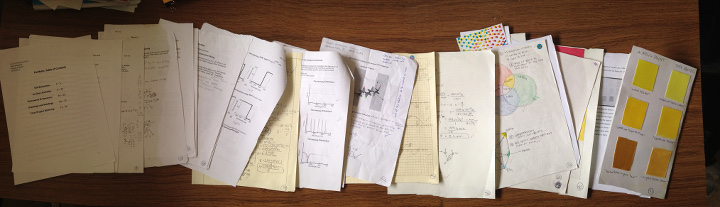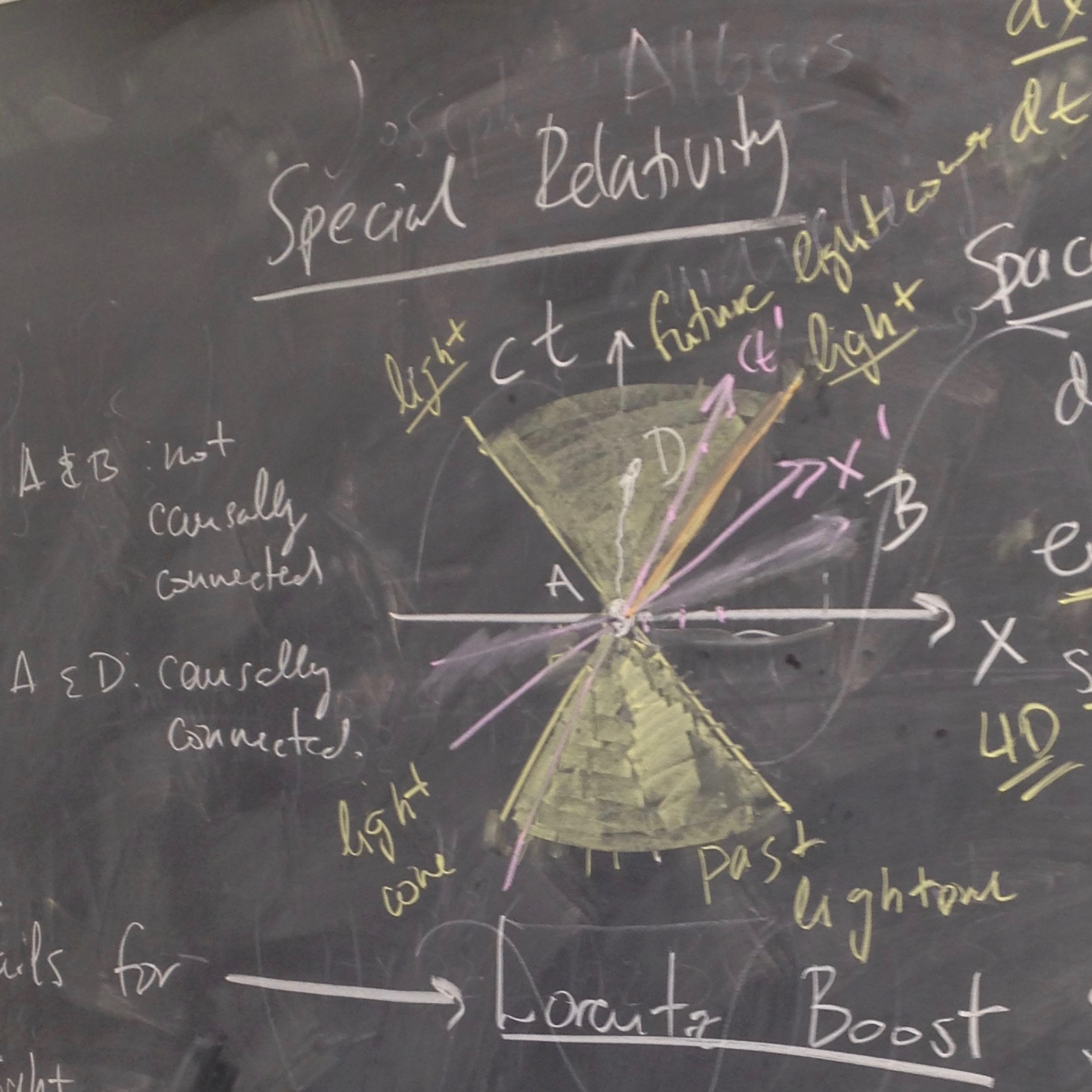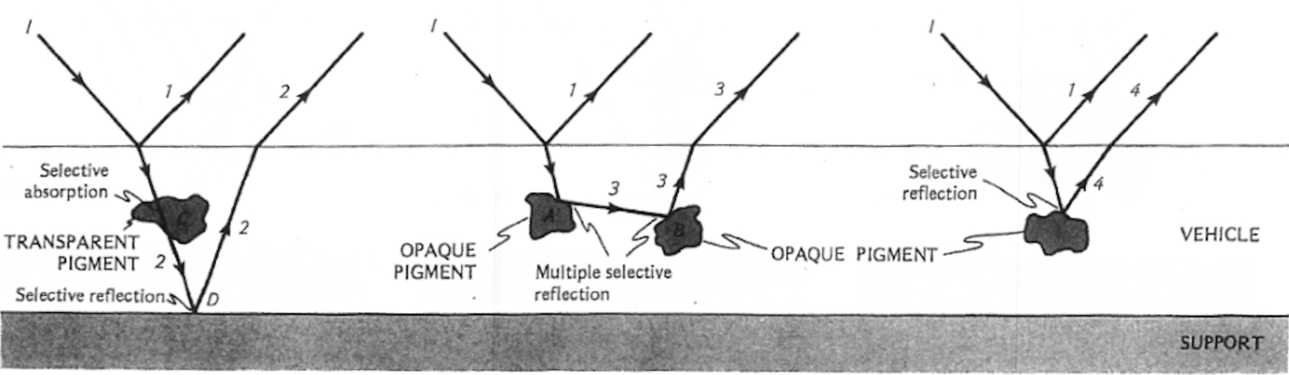## Color Physics @Hampshire, Days 22-23

On Days 22-23, students worked on their individual projects and final portfolios.## Discovering Group Theory @Hampshire, Days 24-27

For the last two weeks of the semester students worked independently on projects which involved the material we learned in class.The class time was spent on research and discussion of their individual projects.

• A second-year pre-med/math student researched the use of group theory in chemistry.
• A first-year student, still undecided on her concentration, dove into a paper on applications of group theory to molecular systems biology and used what we learned in class to understand the key points made in the article (Rietman EA, Karp RL, Tuszynski JA. Review and application of group theory to molecular systems biology. Theoretical Biology & Medical Modelling. 2011;8:21. doi:10.1186/1742-4682-8-21.)
• A first-year art student explored and identified the approximate symmetries of geometric shapes and symbols of ancient South-Western petroglyphs and rock paintings, which included dihedral groups and the translation group.
• A third-year art student researched the connections between group theory and the tree branching patterns.
• A third-year student with a concentration in math researched the application of group theory to Rubik’s cube.

The course concluded with class presentations.

After I complete narrative evaluations for the students in the course, I will write a post reflecting on my own experience teaching this course.

## Discovering Group Theory, Days 21-23

We spent three days discussing the conceptual framework of special relativity.

We reviewed the expressions for time dilation and length contraction (we didn’t derive the latter) and discussed what they tell us about the nature of space and time.  We introduced the Lorentz boosts as a replacement for the Galilean boosts, and formally introduced the Lorentz group, SO(1,3). New concepts and ideas included

• Spacetime events
• Spacetime diagrams and null cones
• Causally connected events
• Relativity of simultaneity

The key takeaway was that Lorentz transformations leave the causal structure of spacetime the same, just like rotations of a polygon leave the shape and the orientation of the polygon the same.## Discovering Group Theory, Day 20

On Day 20, after reviewing the Galilean group and the classic formula for the addition of velocities, we went on to talk about their incompatibility with the results of the Michelson–Morley experiment. We begun to resolve the issue by deriving the expression for time dilation using the example of a moving light clock.

## Color Physics @Hampshire, Day 21

On Day 21, we first reviewed the interaction of light and matter. Students then used hand-held spectrometers to observe and record the spectrum of hydrogen and identified the mystery gas in one of the tubes.

## Color Physics @Hampshire, Day 20

On Day 20, we covered two different topics.

We first discussed how reflection and refraction affect the saturation of pigments.Reflection and Refraction in pigments from Seeing the Light: Optics in Nature, Photography, Color, Vision, and Holography by David R. Falk, Dieter R. Brill , David G. Stork. Wiley, 1986.

We then moved on to some basic quantum mechanics in order to explain why various pigments absorb and reflect different wavelengths. We used a simple model of atom energy levels of sodium. New concepts introduced included

• Photons and their energy E=hc/λ
• Quantized atomic energy levels
• Energy levels of sodium
• Emission and absorption spectra of gases

## Color Physics @Hampshire, Day 19

On Day 19 we studied reflection and refraction. Students used light sticks, mirrors, and acrylic blocks to investigate, both qualitatively and quantitatively, the optical behavior of light at the interface of material with different indices of refraction. Their investigations led us to the law of reflection and Snell’s law.*

We introduced a number of new concepts:

• index of refraction
• normal to the surface
• angle of incidence
• angle of reflection
• angle of refraction/transmitted angle

*The photgraphs taken by and used with the permission of Andrew Hart of Hampshire College.

## Color Physics @Hampshire, Day 18

On Day 18 we

• Discussed the pieces students drew/painted over the course of the last three daysA halftone exercise with watercolors by Madeleine Perreault.

• Practiced how to determine the spectral composition of reflected light from the spectral composition of incident light and the reflectance curves of the surfaces.Given date for a sample problem for which students used the incident intensity distribution and the reflectance curve to find the intensity distribution of the reflected light.

## Discovering Group Theory, Day 19

For this portion of the course, my key goal is that students understand  how group theory is used in physics, so that they can pursue the subject further if it catches their interest.

In preparation for day 19, students were asked to work through Khan Academy videos on 2×2 matrices outside of class

For most students, this was a review, but some of them have not seen matrices before. Rather than introducing everything about matrices, we only discussed those operations needed to prove that rotation matrices form a group SO(2) under matrix multiplication.

While proving that the set of 2×2 rotation matrices is closed under matrix multiplication, we used our recently acquired knowledge of complex numbers to derive the equations for cosine and sine of the sum of the angles.We introduced SO(3) by analogy to SO(2), but without going into the technical details.

After wrapping up rotations, we then moved on to moving frames of reference and the Galilean principle of relativity. We showed that acceleration is the same in all frames of reference moving at constant velocity relative to one another. This calculation required the understanding of a derivative, which was familiar to most students but not all, so I emphasized the conceptual understanding. We then defined Galilean group.